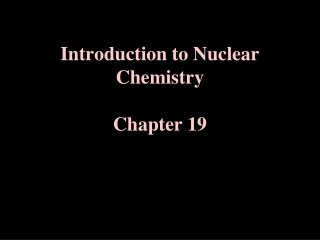DownloadDownload PresentationIntroduction to Nuclear Chemistry Chapter 19

# Introduction to Nuclear Chemistry Chapter 19

Télécharger la présentation## Introduction to Nuclear Chemistry Chapter 19

- - - - - - - - - - - - - - - - - - - - - - - - - - - E N D - - - - - - - - - - - - - - - - - - - - - - - - - - -
##### Presentation Transcript

1. Introduction to Nuclear ChemistryChapter 19

2. I. Study of Nuclear Reactions Nuclear Reactions vs. Chemical Reactions Define “ Chemical Reaction”?

3. Define “Nuclear Reaction” Reaction involving spontaneous emission of radiation by an unstable nuclei to a more stable nuclei of a different element. Involves changes within nucleus. Atoms of each element do not balance. Spontaneous change of a radioisotope.

4. Example Nuclear Reactions:

5. Rules For Balancing Nuclear Reactions: 1. The sum of the mass numbers of the reactants must equal the sum of the mass number of the products. *Total # nucleons remain the same. 2. The sum of the atomic numbers of the reactants must equal the sum of the mass number of the products. * Electrical charge remains the same.

6. III. Radioactive Decay Rates Follows First Order Kinetics Commonly described by “half-life”. t1/2 “The time required for half the radioactive atoms in a sample to undergo decay.”

7. Derived From Integrated Rate Law:

8. Examples of Half-Lives

9. Iodine-131 (t1/2 = 8 days)

10. Example Problem: The value of t1/2 for I-131 is 8 days. How many I-131 atoms will remain after 24 days in a sample originally containing 6.4 x 1016 I-131 atoms?